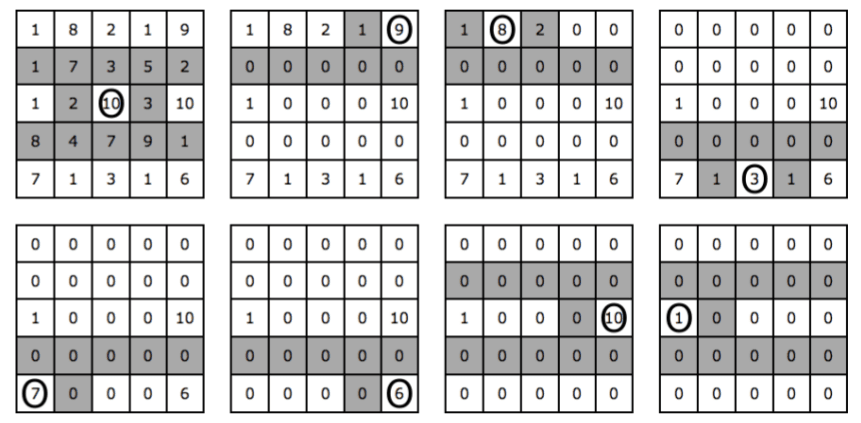C - Candy

Languages: C, C++, Java, JavaScript, ... (details)

Little Charlie is a nice boy addicted to candies. He is even a subscriber to All Candies Magazine and was selected to participate in the International Candy Picking Contest.

In this contest a random number of boxes containing candies are disposed in $M$ rows with $N$ columns each (so, there are a total of $M \times N$ boxes). Each box has a number indicating how many candies it contains.

The contestant can pick a box (any one) and get all the candies it contains. But there is a catch (there is always a catch): when choosing a box, all the boxes from the rows immediately above and immediately below are emptied, as well as the box to the left and the box to the right of the chosen box. The contestant continues to pick a box until there are no candies left.

The figure bellow illustrates this, step by step. Each cell represents one box and the number of candies it contains. At each step, the chosen box is circled and the shaded cells represent the boxes that will be emptied. After eight steps the game is over and Charlie picked $10 + 9 + 8 + 3 + 7 + 6 + 10 + 1 = 54$ candies.For small values of $M$ and $N$, Charlie can easily find the maximum number of candies he can pick, but when the numbers are really large he gets completely lost. Can you help Charlie maximize the number of candies he can pick?

Input

The input contains several test cases. The first line of a test case contains two positive integers $M$ and $N$ $(1 \leq M \times N \leq 10^5)$, separated by a single space, indicating the number of rows and columns respectively. Each of the following $M$ lines contains $N$ integers separated by single spaces, each representing the initial number of candies in the corresponding box. Each box will have initially at least $1$ and at most $10^3$ candies.

The end of input is indicated by a line containing two zeroes separated by a single space.

The input must be read from standard input.

Output

For each test case in the input, your program must print a single line, containing a single value, the integer indicating the maximum number of candies that Charlie can pick.

The output must be written to standard output.

Sample test(s)

Input
5 5 1 8 2 1 9 1 7 3 5 2 1 2 10 3 10 8 4 7 9 1 7 1 3 1 6 4 4 10 1 1 10 1 1 1 1 1 1 1 1 10 1 1 10 2 4 9 10 2 7 5 1 1 5 0 0
Output
54 40 17